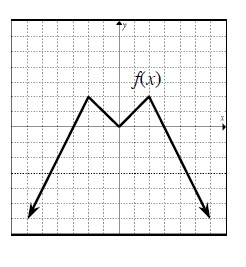### Home > PC > Chapter 2 > Lesson 2.3.6 > Problem2-149

2-149.Given the graph of $f(x)$ to the right, sketch graphs of the following transformations. 2-149 HW eTool (Desmos)

1. $-f(x)+2$

Reflect the curve vertically, then shift it up $2$ units.

2. $y=\frac{1}{2}f(x+2)$$\frac{1}{2}f(x+2)$

Shift the curve left $2$ units, then halve the $y\text{-values}$.

Use the eTool below to complete parts (a) and (b).
Click the link at right for the full version of the eTool:
2-149 HW eTool (Desmos)